## Maximum Based on Multiple Criteria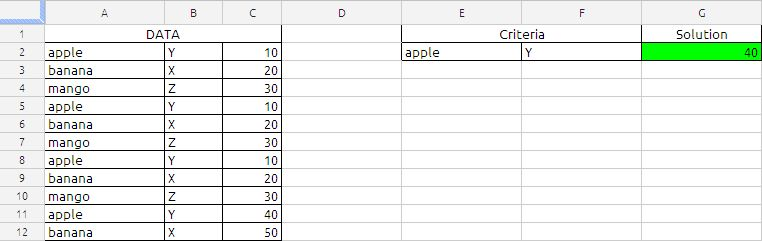The Formula in cell G2 is
=ArrayFormula(MAX(IF(A2:A12=E2,IF(B2:B12=F2,C2:C12))))

the above formula will return the maximum value in cell C2:C12 corresponding to criteria specified in the cell E2 and F2.

the formula will narrows down using the conditions in the IF function first A2:A12 and then B2:B12. If the both conditions are met the resultant will be value in cell C2:C12

Which will be MAX {10,10,10,40}

based on the sample data the result will be 40.

## Net Present Value or NPV

The NPV function returns the net present value of an investment based on a series of potentially irregular cash flows that occur at regular time intervals.

Oxford Dictionary of Accounting defines Net Present Value(NPV) as

“A method of capital budgeting in which the value of an investment is calculated as the total present value of all cash inflows and cash outflows minus the cost of initial investment. If the net present value is positive, the return will be greater than that required by the capital markets and investment should be considered. A negative net present value indicates that the investment should be rejected.”

Example:

A Company is considering the purchase of a new machine, which is expected to save 100,000 in cash operating costs each year. Its estimated useful life(how long it will last) is five years, at the end of which it will have no net residual value.

The cash flows for the project are as follows:

Year 0 : Cost of the machine 390,000

Year 1: Savings of 100,000

Year 2: Savings of 100,000

Year 3: Savings of 100,000

Year 4: Savings of 100,000

Year 5: Savings of 100,000

A simple analysis shows that the savings over five years are 500,000 (5 X 100,000) and the cost is 390,000, which gives a surplus of 110,000. However, such an analysis ignores the time value of money; 1 received in future is not equal to 1 received today. To calculate the present value of future cash flows it is necessary to calculate the discount factor.

Assuming that the cost of capital for the project is 8%, the discount factor is calculated as follows:

 Year 1 1/(1.08)^1 Year 2 1/(1.08)^2 Year 3 1/(1.08)^3 Year 4 1/(1.08)^4 Year 5 1/(1.08)^5

The net present value can then be calculated: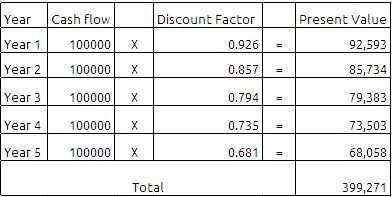If we deduct the initial investment of the machine 390,000 then we will get 9,271 which is positive. so we can accept the investment in the project since it is giving the positive cash flow.

All the above is good to understand the background of the Net Present Value (NPV) as far as finance is concerned.

Now we will see how to use this function in google docs spreadsheets.

The Syntax of the function is as follows:

NPV(Rate, value_1, value_2, … value_30)

Rate: The discount rate per period. periodic-discount-rate is a number value and is either entered as a decimal (for example, 0.08) or with a percent sign (for example, 8%). periodic-discount-rate must be greater than or equal to 0.

Value_1, Value_2, …….Value_3 :Values is a number value. A positive value represents income (cash inflow). A negative value represents an expenditure (cash outflow). Value must be equally spaced in time.

Value_1………..: Optionally include one or more additional cash flows.

Usage Notes

periodic-discount-rate is specified using the same time frame as the time frame used for the cash flows. For example, if the cash flows are monthly and the desired annual discount rate is 8%, periodic-discount-rate must be specified as 0.00667 or 0.667% (0.08 divided by 12).

If cash flows are irregular, use the IRR function.

We can achieve the same results using the NPV function as shown in the below image: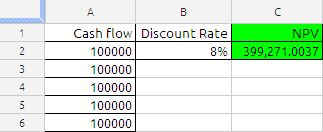The formula in cell C2 is

=NPV(B2,A2:A6)

## unique random number between 1,10 in single array formula

The Randbetween function may generate duplicate value. if we used the formula

=Randbetween(1,10) it will generate random numbers between 1 and 10, if we applied the formula in ten cells there is a possibility the formula may generate duplicate value in the ten cells. To avoid this you can use the following formula

=ARRAYFORMULA(LARGE(ROW(\$1:\$10)*NOT(COUNTIF(\$A\$1:A1, ROW(\$1:\$10))), RANDBETWEEN(1,11-ROW(A1))))

Copy cell A2 and paste down as far as needed.

### Explaining the alternative array formula in cell A2

Step 1 – Create an array
ROW(\$1:\$10) creates this array {1, 2, 3, 4, 5, 6, 7, 8, 9, 10}

Step 2 – Create a criterion to avoid duplicate numbers
COUNTIF(\$A\$1:A1, ROW(\$1:\$10)) makes sure no duplicate numbers are created. The formula has both absolute and relative cell references (\$A\$1:A1). When the formula are copied down to cell A3 the cell reference changes to \$A\$1:A2. The value in cell A2 can´t be randomly selected again.
In cell A2, COUNTIF(\$A\$1:A1, ROW(\$1:\$10)) creates this array: {0, 0, 0, 0, 0, 0, 0, 0, 0, 0}

Step 3 – Create a new dynamic array
ROW(\$1:\$10)*NOT(COUNTIF(\$A\$1:A1, ROW(\$1:\$10))) creates this array in cell A2: {1, 2, 3, 4, 5, 6, 7, 8, 9, 10}
If the array formula  randomly selects the number 2 in cell A2, the formula in cell A3 creates this array: {1, 0, 3, 4, 5, 6, 7, 8, 9, 10}
Number 2 can´t be selected anymore.

Step 4 – Calculate the number range in Randbetween(bottom, top)
The bottom value is always 1. The top value changes depending on current cell.
In cell A2 the top value is 10.
In cell A3 the top value is 9
and so on..
Formula in cell A2: 11-ROW(A1) equals 10. (11-1=10)
Formula in cell A3: 11-ROW(A2) equals 9. (11-2=9)
and so on..

Step 5 – Create a random number
=LARGE(ROW(\$1:\$10)*NOT(COUNTIF(\$A\$1:A1, ROW(\$1:\$10))), RANDBETWEEN(1,11-ROW(A1)))
RANDBETWEEN(1,11-ROW(A1))
becomes
RANDBETWEEN(1,11-1)
becomes
RANDBETWEEN(1,10)
and returns a random number between 1 and 10.

Step 6 – Select a random number in array
=LARGE(ROW(\$1:\$10)*NOT(COUNTIF(\$A\$1:A1, ROW(\$1:\$10))), RANDBETWEEN(1,11-ROW(A1)))
becomes
=LARGE({1, 2, 3, 4, 5, 6, 7, 8, 9, 10}, RANDBETWEEN(1,10))
becomes
=LARGE({1, 2, 3, 4, 5, 6, 7, 8, 9, 10}, random_number) and returns a random number between 1 and 10.

## SUMPRODUCT

The SUMPRODUCT function returns the sum of the products of corresponding numbers in one or more arrays.

SUMPRODUCT (array 1, array 2, …array 30)

array1: A array  of cells. array 1  is a reference to a single range of cells containing values of any type. If string or Boolean values
array 2,……. array 30: Optionally include one or more additional array ( ranges) of cells. The ranges must all have the same dimensions.

Usage Notes
The SUMPRODUCT function multiplies the corresponding numbers in each range and then sums each of the products. If only one range is specified, SUMPRODUCT returns the sum of the range.

## Traditional Use of SUMPRODUCT

In it’s traditional form or classic form, SUMPRODUCT multiplies each value in one array by the corresponding value in another array, and returns the summed result. As an example, if cells A1:A3 contain the values 1,2,3 and B1:B3 contain 10,20,30, then

=SUMPRODUCT(A1:A3,B1:B3)

returns 140, or (1*10)+(2*20)+(3*30)=10+40+90=140.

This is a useful function, but nothing more than that. A further, more ‘creative’ use of SUMPRODUCT has evolved, and is still evolving. This has been a creative and productive process that has significantly increased the useability of SUMPRODUCT,

## Advanced Use of SUMPRODUCT

There are two very useful functions that support conditional counting and summing, namely COUNTIF and SUMIF. Very useful functions, but limitation in that they can only evaluate a single test range.

To know more about the countif function have look at the following link
https://anandexcels.wordpress.com/2012/01/28/countif/

To know more about the Sumif have look at the following link
https://anandexcels.wordpress.com/2012/01/28/sumif-function/

The only constrain in these function is it will evaluate a single criteria, all thou we can force them to do multiple criteria using IF function

We will look into more rational way of forcing he sumproduct function to do more using a array formula. if you observe in the functions list it is under the array formula’s group. lets try to understand the functionality using an example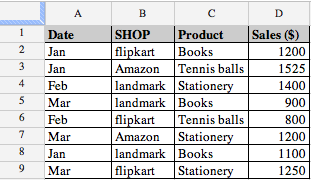We can easily count the number of Amazon with
=countif(B2:B9,”Amazon”)
which returns 2.
Similalrly, it is straight-forward to get the value of products sold through “Amazon”, using
=SUMIF(B2:B9,”Amazon”,D2:D9)
which gives 2725.
But supposing that we want a count of how many Amazon  sold in Jan, or the value of them? The number can be calculated with
=ArrayFormula(SUM(IF(B2:B9=”Amazon”,IF(A2:A9=”Jan”,1,0),0)))
which is an array formula. Similarly, the value is obtained with
=ArrayFormula(SUM(IF(B2:B9=”Amazon”,IF(A2:A9=”Jan”,D2:D9,0),0)))
which is also an array formula.

But as this page is about SUMPRODUCT, you would expect that we could use that function in this case, and we can. The solution for the number of Amazon sold in Jan using this function is
=ArrayFormula(SUMPRODUCT((B2:B9=”Amazon”)*(A2:A9=”Jan”)))
The value is obtained with
=ArrayFormula(SUMPRODUCT((B2:B9=”Amazon”)*(A2:A9=”Jan”)*(D2:D9)))
The * is being used as the AND operator, the formula is saying, where A2:A9 = Amazon AND B2:B9 = Jan, and where A2:A9 = Amazon AND B2:B9= Jan, multiplied by D2:D9.

This is relatively easy to use when there are multiple criteria.

We can see that the * is equivalent to AND in the formula, how this works is explained later, but supposing we want an OR condition. As a further extension of its use, we use the ‘+’ (plus) operator to count OR conditions, such as how many books sold were either
Flipkart or Landmark. The formula for this is
=ArrayFormula(SUMPRODUCT((B2:B9=”Flipkart”)+(B2:B9=”landmark”)))
which returns the result 6 as expected
So far, so good, in that we have a versatile function that can do any number of conditional tests, and has an inbuilt flexibility that provides extensibility.

## SUMPRODUCT Explained

Understanding how SUMPRODUCT works helps to determine where to use it, how to can construct thus formula, and thus how it can be extended. below shows an example data set that we will use.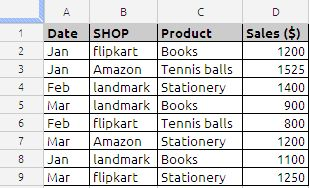In this example, the problem is to find how many Amazon with a date in Jan were sold. A2:A9 holds the Date, B2:B9 has the Shop, and D2:D9 has the number sold. The formula to get this result is
=ArrayFormula(SUMPRODUCT((B2:B9=”Amazon”)*(A2:A9=”Jan”)*(D2:D9)))
The first part of the formula (B2:B9=”Amazon”) checks the array of makes for a value of Amazon. This returns an array of TRUE/FALSE, in this case it is
{FALSE,TRUE,FALSE,FALSE,FALSE,TRUE,FALSE,FALSE}
Similarly, the categories are checked for the vale Date with (A2:A9=”Jan”). Again, this returns an array of TRUE/FALSE, or
{TRUE,TRUE,FALSE,FALSE,FALSE,FALSE,TRUE,FALSE}
And finally, the numbers are not checked but taken as is, that is (D2:D9), which returns an array of numbers
{1200,1525,1400,900,800,1200,1100,1250}
So now we have three arrays, two of TRUE/FALSE values, one of numbers. This is showm in below.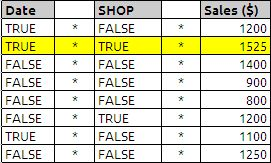And this is where it gets interesting.

SUMPRODUCT usually works on arrays of numbers, but we have arrays of TRUE/FALSE values as well as an array of numbers. By using the ‘*’ (multiply) operator, we can get numeric values that can be summed. ‘*’ has the effect of coercing these two arrays into a single array of 1/0 values. Multiplying TRUE by TRUE returns 1 (try it, enter =TRUE()*TRUE() in a cell and see the result), any other combination returns 0. Therefore, when both conditions are satisfied, we get a 1, whereas if any or both conditions are not satisfied, we get a 0. Multiplying the first array of TRUE/FALSE values by the second array of TRUE/FALSE values returns a composite array of 1/0 values, or

{0,1,0,0,0,0,0,0}*{1200,1525,1400,900,800,1200,1100,1250}
which will result in 1525

Similarly we can count the same
=ArrayFormula(SUMPRODUCT((B2:B9=”Amazon”)*(A2:A9=”Jan”)))
the arrays will be as show in the below example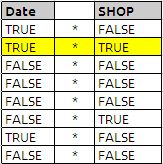and after executing the above formulas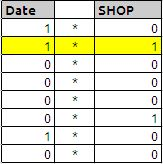and answer is highlighted in yellow colour which will result in value 1

If you have been able to follow this explanation all of the way through, it may have occurred to you that although we are using the SUMPRODUCT function, the ‘*’ operators have resolved the multiple arrays into a single composite array, leaving SUMPRODUCT to simply sum the members of that composite array, that is, there is no product. This is perfectly correct, and perfectly valid,SUMPRODUCT can work on a single array (put 1,2,3 in cells A1,A2,A3, and insert =SUMPRODUCT(A1:A3) in a cell, it returns 6 correctly). In reality, we only need the ‘*’ to coerce the arrays that are being tested for a particular condition, we do not need it for the array that is not subject to a conditional test. So we could also use

=ArrayFormula(SUMPRODUCT((B2:B9=”Amazon”)*(A2:A9=”Jan”)*(D2:D9)))

which does use the product aspect .

• Double uninary will not work in google docs spead sheets.
• When using the SUMPRODUCT function, all arrays must be the same size, as corresponding members of each array are multiplied by each other.
• When using the SUMPRODUCT function, do not use the open ranges it may cause errors

In a SUMPRODUCT function, the arrays being evaluated cannot be a mix of column and row ranges, they must all be columns, or all rows. However, the row data can be transposed to present it to SUMPRODUCT as columnar.

## More about Dates

We are looking into some more Weekday formula in addition to my earlier blog post about Weekday function.

In this example we are identifying  the first and last weekdays using the Weekday, Month, Date & MOD function in Google Docs Speadsheets.

The Chosen date is in cell B1,

the link to my earlier Weekday function

## ROUNDING

Rounding is mainly used in financial transactions which involve currency, payroll processing, invoicing, taxation, financial projections. many people do not use the rounding in Budgeting because this does not involves any financial  implication. personally i preferes to use this rounding when every money is involved.

Now we will see what are the rules of rounding as per Mathematics

Rules for Rounding off numbers.

In approximate computations it is frequently necessary to round off numbers (both approximate and exact), which means dropping one or more of the last digits. To ensure that the rounded number is as close as possible to the original number, use the following rules.

Rule 1. If the first of the discarded  digits exceeds 5, then the last digit kept is increased by unity. The increase is also made when the first digit kept is equal to 5 and is followed by one or more significant digits (for the case when the discarded 5 is not followed  by any digits, see Rule 3 below)

Rule 2. If the first digit dropped is less than 5, no increase is made

Rule 3. If the digit 5 is dropped, and no significant digits come after it, the rounding is done to the closest even number; that is the last retained digit is left unchanged if it is odd. The reason for this rule is given below.

Ex 1: Rounding 0.0465 to the third decimal place, we write 0.046. We do not increase the last digit kept since it is even. The number 0.046 is just as close to the given one as is 0.047

Ex 2: Rounding 0.935 to the second decimal place, we write 0.94. the last retained digit 3 is increased because it is odd.

Ex 3: Rounding the numbers, 6.527, 0.456, 2.195, 1.450, 0.950, 4.851, 0.850, 0.05 to the first decimal place, we get 6.5, 0.5, 2.2, 1.4, 1.0, 4.9, 0.8, 0.0

Note: When applying Rule 3 to the rounding off of one number do not increase the accuracy of the rounding process (Ex 1 and 2). However, if the process is performed repeatedly, there will be roughly just as many numbers with excess as with deficit. The mutual compensation of errors will ensure the highest possible accuracy  of the result.

Rule 3 can be modified and made to apply to rounding off to the closest odd number. The accuracy will be the same but even digits are more convenient than odd numbers.

In the spread sheet:

Standard Rounding Rules:

1) select position you want to round to;

2) look 1 to the right and if that is 5 or greater, add 1; if it is 4 or less, don’t add 1; then remove all other digits.

The ROUND function follows the standard rounding rules and rounds to a specified digit

The ROUNDUP function always rounds up to a specified digit

The ROUNDDOWN function always rounds down to a specified digit

The MROUND function follows the standard rounding rules and rounds to a specified amount (multiple)

The CEILING function always rounds up to a specified amount (multiple)

The FLOOR function always rounds down to a specified amount (multiple)

Now lets see each function in details with their syntax and examples.

(1) The ROUND function returns a number rounded to the specified number of places.

The Syntax of the function is as follows:

ROUND(number, count)

number:  The number to be rounded. number is a number value.

count:  The number of digits you want to retain, relative to the decimal point. digits is a number value. A positive number represents digits (decimal places) to the right of the decimal point to include. A negative number specifies digits to the left of the decimal point to replace with zeros (the number of zeros at the end of the number).

All ways remember Decimal place ( . ) is significant in the round function. If the number argument is 2 then value spit out by the function will have upto two decimals. if the number is 0 then the only integer part will only will come. if the number argument is -2 then the number is rounded to nearest hundred this you can clearly see the following picture.

Example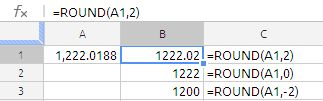(2) MROUND: The MROUND function rounds a number to the nearest multiple of a specified factor.

The Syntax of the function is as follows

MROUND(number, multiple)

number:  The number to be rounded. num-to-round is a number value.

multiple:  The number to use to determine the closet multiple. multiple is a number

value. It must have the same sign as number.

Examples:

MROUND(12.0188,5) returns 10

MROUND(12.7187,5) return 15

MROUND(14.99,5) return 15

MROUND(15.01,5) return 15

MROUND(17.6,5) return 20

(3)ROUNDUP :function returns a number rounded away from zero (rounded up) to the specified number of places.

The Syntax of the function is as follows:

ROUNDUP(number, count)

number:  The number to be rounded. number is a number value.

count:  The number of digits you want to retain, relative to the decimal point. digits is a number value. A positive number represents digits (decimal places) to the right of the decimal point to include. A negative number specifies digits to the left of the decimal point to replace with zeros (the number of zeros at the end of the number).

Example(4) ROUNDDOWN: The ROUNDDOWN function returns a number rounded toward zero (rounded down) to the specified number of places.

The Syntax of the functions is

ROUNDDOWN(number, count)

number:  The number to be rounded. number is a number value.

count:  The number of digits you want to retain, relative to the decimal point. digits is a number value. A positive number represents digits (decimal places) to the right of the decimal point to include. A negative number specifies digits to the left of the decimal point to replace with zeros (the number of zeros at the end of the number).

Example: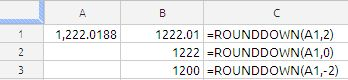(5) INT function: Some times INT is very useful to round the numbers

The INT function returns the nearest integer that is less than or equal to the number.

the syntax of the function is as follows:

INT(number)

number:  The number to be rounded. number is a number value.

Example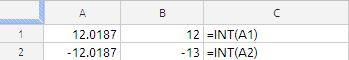(6) FLOOR :The FLOOR function rounds a number toward zero to the nearest multiple of the specified factor.

The Syntax of the function is as follows:

FLOOR(number, significance, mode)

number:  The number to be rounded. number is a number value.

significance:  The number to use to determine the closet multiple. factor is a number value. It must have the same sign as number.

mode: Mode is an optional value. If it is indicated and non-zero and if the number and significance are negative, rounding up is carried out based on that value.

CEILING: The CEILING function rounds a number away from zero to the nearest multiple of the specified factor.

The Syntax of the function is

CEILING(number, significance, mode)

number:  The number to be rounded. num-to-round is a number value.

significance:  The number to use to determine the closet multiple. multiple-factor is a number value and must have the same sign as num-to-round.

mode: Mode is an optional value. If it is indicated and non-zero and if the number and significance are negative, rounding up is carried out based on that value.

Example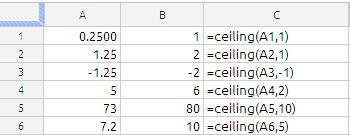TRUNC : The TRUNC function truncates a number to the specified number of digits.

Syntax of the function is as follows:

TRUNC(number, count)

number:  A number. number is a number value.

count:  An optional value specifying the number of digits you want to retain, relative to the decimal point. count is a number value. A positive number represents digits (decimal places) to the right of the decimal point to include. A negative number specifies digits to the left of the decimal point to replace with zeros (the number of zeros at the end of the number).

example: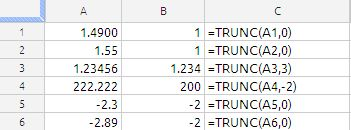Example of all functions combines on same data set.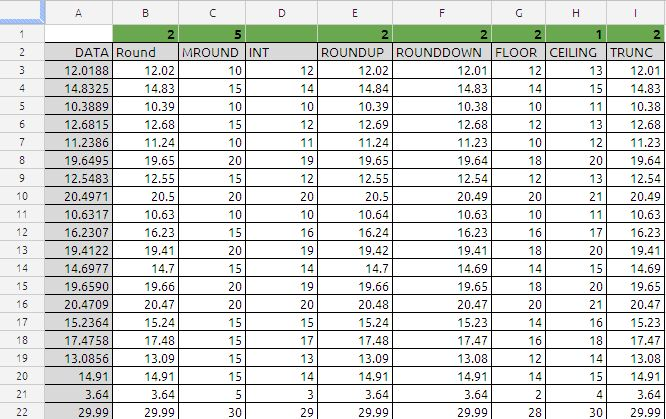In cell A2 to A22 are the data set and in cell B1 TO I1 are the second arguments of the each function except column D which INT which is single argument function.

you can down load the above spread sheet at the following link